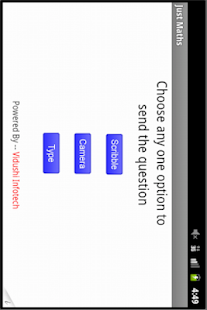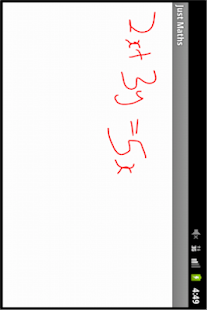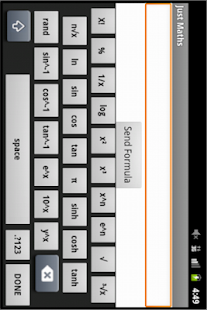JustMath The application includes a powerful expression interpreter which is able to solve all your expressions. The program interface makes it easy to edit the expression, plot functions

Internet access is required only for error reporting.

Features

• Basic arithmetic operations: + – * /
• The square root
• Exponentiation
• Trigonometric functions
• Logarithmic functions
• Graphs
• Solution of the equations
• Cycles for / each
• Arrays

Screenshots

•••